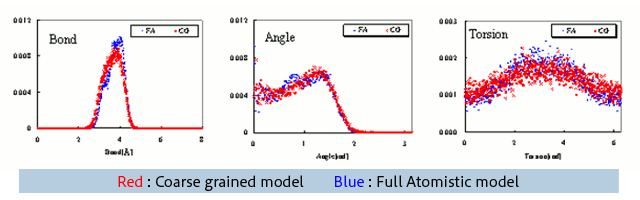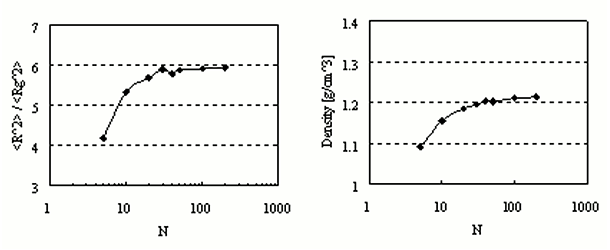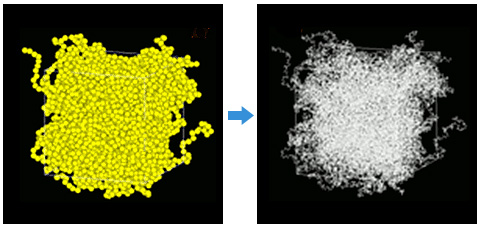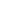Full Atomistic MD
Coarse Grained MD
Multiscale Analysis
Other Properties
Materials Science

# Evaluation of Coarse Grained Potential.

The Coarse Grained MD of polybutadiene were evaluated. The Coarse Grained Potential was estimated using the modeling functions in J-OCTA. The method follows that given in Reference (1). First, the MD were simulated for a single chain in a vacuum (total time=10 [ns]) for cis-polybutadiene (N=50) based on the full atomistic model.

Using the calculation results, the distributions of bond lengths, bond angles, and dihedral angles between the coarse grained units were evaluated, and the Coarse Grained Potential was determined from the obtained distributions. For the non-bonded potential, the interaction between the coarse grained units was evaluated directly.

The distributions of bond lengths, bond angles, and dihedral angles between coarse grained units are shown in Fig. 1. The results from the full atomistic model and the coarse grained model are in good agreement. Here in Coarse Grained MD, the calculation of the bond dihedral angle potential was omitted due to calculation stability problems, but it is evident that the effect is small in this example.Fig.1 Distribution of length, angle, and torsion angle. (Unit = one monomer)
(Red = Coarse grained model, Blue = Full Atomistic model)

NPT ensemble calculations were conducted at 300 K and 0.1 MPa for each bulk state with different degrees of polymerization N. The total number of particles was the same, 5000, and long-term simulation (400 ns) was used for the system with N=200.

The ratio of the mean square of the radius of gyration (Rg) to the end-to-end distance converges to six as N increases (Fig. 2, left).Fig.2 R^2/Rg^2 and density at bulk state.

Finally, a bulk structure of the full atomistic MD was created using J-OCTA's reverse mapping function (Fig. 3). From the results of Coarse Grained MD, the monomers of the full atomistic model corresponding to each coarse grained unit were arranged and connected so that the direction of each bond vector matched. This process allows the creation of a more relaxed structure of the full atomistic model.Fig.3 Reverse mapping (left = coarse grained model, right = full atomistic model)

• Reference
• (1) J. Chem. Phys., 116, 8183, (2002)
• (2) Macromolecules, 42, 791, (2009)This website uses cookies to improve functionality and performance. If you continue browsing the site, you are giving implied consent to the use of cookies on this website. If you want to know more or refuse consent, read our Cookie Policy.

Accept